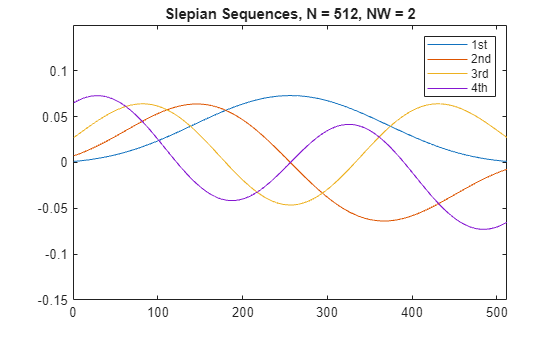Documentation

### This is machine translation

Mouseover text to see original. Click the button below to return to the English version of the page.

# dpss

Discrete prolate spheroidal (Slepian) sequences

## Syntax

```dps_seq = dpss(seq_length,time_halfbandwidth) [dps_seq,lambda] = dpss(seq_length,time_halfbandwidth) [...] = dpss(seq_length,time_halfbandwidth,num_seq) [...] = dpss(seq_length,time_halfbandwidth,'interp_method') [...] = dpss(...,Ni) [...] = dpss(...,'trace') ```

## Description

`dps_seq = dpss(seq_length,time_halfbandwidth)` returns the first `round(2*time_halfbandwidth)` discrete prolate spheroidal (DPSS), or Slepian sequences of length `seq_length`. `dps_seq` is a matrix with `seq_length` rows and `round(2*time_halfbandwidth)` columns. `time_halfbandwidth` must be strictly less than `seq_length/2`.

`[dps_seq,lambda] = dpss(seq_length,time_halfbandwidth)` returns the frequency-domain energy concentration ratios of the column vectors in `dps_seq`. The ratios represent the amount of energy in the passband [–W,W] to the total energy from [–Fs/2,Fs/2], where Fs is the sample rate. `lambda` is a column vector equal in length to the number of Slepian sequences.

`[...] = dpss(seq_length,time_halfbandwidth,num_seq)` returns the first `num_seq` Slepian sequences with time half bandwidth product `time_halfbandwidth` ordered by their energy concentration ratios. If `num_seq` is a two-element vector, the returned Slepian sequences range from `num_seq(1)` to `num_seq(2)`.

`[...] = dpss(seq_length,time_halfbandwidth,'interp_method')` uses interpolation to compute the DPSSs from a user-created database of DPSSs. Create the database of DPSSs with `dpsssave` and ensure that the resulting file, `dpss.mat`, is in the MATLAB® search path. Valid options for `'interp_method'` are `'spline'` and `'linear'`. The interpolation method uses the Slepian sequences in the database with time half bandwidth product `time_halfbandwidth` and length closest to `seq_length`.

`[...] = dpss(...,Ni)` interpolates from DPSSs of length `Ni` in the database dpss.mat.

`[...] = dpss(...,'trace')` prints the method used to compute the DPSSs in the command window. Possible methods include: direct, spline interpolation, and linear interpolation.

## Examples

collapse all

Construct the first four discrete prolate spheroidal sequences of length 512. Specify a time half bandwidth product of 2.5. Plot the sequences and find the concentration ratios.

```seq_length = 512; time_halfbandwidth = 2.5; num_seq = 2*(2.5)-1; [dps_seq,lambda] = dpss(seq_length,time_halfbandwidth,num_seq); plot(dps_seq) title('Slepian Sequences, N = 512, NW = 2.5') axis([0 512 -0.15 0.15]) legend('1st','2nd','3rd','4th')````concentration_ratios = lambda'`
```concentration_ratios = 1×4 1.0000 0.9998 0.9962 0.9521 ```

collapse all

### Discrete Prolate Spheroidal Sequences

The discrete prolate spheroidal or Slepian sequences derive from the following time-frequency concentration problem. For all finite-energy sequences $x\left[n\right]$ index limited to some set $\left[{N}_{1},{N}_{1}+{N}_{2}\right]$, which sequence maximizes the following ratio:

`$\lambda =\frac{\underset{-W}{\overset{W}{\int }}|X\left(f\right){|}^{2}df}{\underset{-Fs/2}{\overset{Fs/2}{\int }}|X\left(f\right){|}^{2}df}$`

where Fs is the sample rate and $|W|. Accordingly, this ratio determines which index-limited sequence has the largest proportion of its energy in the band [–W,W]. For index-limited sequences, the ratio must satisfy the inequality $0<\lambda <1$. The sequence maximizing the ratio is the first discrete prolate spheroidal or Slepian sequence. The second Slepian sequence maximizes the ratio and is orthogonal to the first Slepian sequence. The third Slepian sequence maximizes the ratio of integrals and is orthogonal to both the first and second Slepian sequences. Continuing in this way, the Slepian sequences form an orthogonal set of bandlimited sequences.

### Time Half Bandwidth Product

The time half bandwidth product is NW where N is the length of the sequence and [–W,W] is the effective bandwidth of the sequence. In constructing Slepian sequences, you choose the desired sequence length and bandwidth 2W. Both the sequence length and bandwidth affect how many Slepian sequences have concentration ratios near one. As a rule, there are 2NW – 1 Slepian sequences with energy concentration ratios approximately equal to one. Beyond 2NW – 1 Slepian sequences, the concentration ratios begin to approach zero. Common choices for the time half bandwidth product are: 2.5, 3, 3.5, and 4.

You can specify the bandwidth of the Slepian sequences in Hz by defining the time half bandwidth product as NW/Fs, where Fs is the sample rate.

## References

Percival, D. B., and A. T. Walden. Spectral Analysis for Physical Applications. Cambridge, UK: Cambridge University Press, 1993.